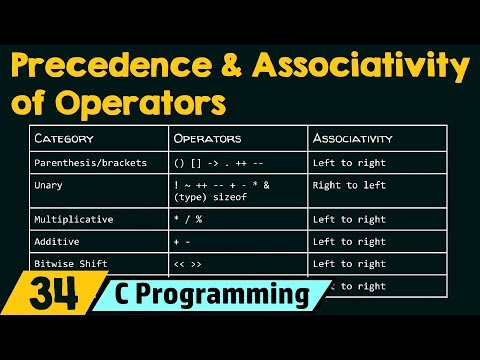If two or more operator is used in an expression, then you should know about precedence and associativity of an operator. The operator precedence in C defines priority. That means which operator should apply first on the given expression. The higher precedence operator evaluates first. But the problem occurs when the operator of the same priority is available in the expression. C Standard introduced a new term to resolve this issue. It is called associativity. The associativity defines the order. In which operators of the same priority are evaluated,  from right to left or left to right.

Operator precedence in C is decreased from top to bottom in below mention table.

 Operator Description of Operator Associativity () Parentheses or Function call Left to right [] Brackets or array subscript Left to right . Dot or member selection operator Left to right -> Arrow operator Left to right ++ -- Postfix increment Postfix decrement Left to right ++ -- Prefix increment Prefix decrement Right to left + - Unary plus Unary minus Right to left ! Not operator Right to left ~ Bitwise complement operator Right to left type Typecast Right to left * Indirection or dereference operator Right to left & Address of operator Right to left Sizeof Determine size in bytes Right to left */% Multiplication, division and modulus Left to right + - Addition Subtraction Left to right << >> Bitwise left shift and right shift Left to right < <= >>= Relational less than or less than equal to Relational greater than or greater than equal to Left to right Left to right == != Relational equal to or not equal to Left to right && Bitwise AND Left to right ^ Bitwise Exclusive OR Left to right | Bitwise Inclusive OR Left to right && Logical AND Left to right || Logical OR Left to Right ?: Ternary operator Right to Left = +=          -= *=          /= %=        &= ^=         |= <<=       >>= Assignment operator Addition/subtraction assignment Multiplication/division assignment Modulus /Bitwise assignment Bitwise exclusive/inclusive OR assignment Right to left , Comma Operator Left to right

In the above mention table, the precedence of the operator is decreased from top to bottom. The operator has higher precedence at the top and lowers precedence at the bottom. Let's understand the operator precedence in C with the help of an example.

### Example 1

Evaluate 10 + 2 *3
It is clear from the operator precedence and associativity table, multiplication operator(*) has higher priority than addition operator (+). So, 2*3 evaluates first,

Now, the addition operator performs; the result will be 16.

10 + 2 * 3 is calculated as 10+(2*3), not like (10+2)*3

### Example 2

Evaluate expression of 100 + 12 / 2 - 45.

In this expression, the division operator (/) has a higher priority. Precedence of the addition (+) and subtraction (-) operator is low.

In this expression, the division operator (/) has higher precedence. So, 12/2 evaluated first. Operator (addition/subtraction) precedence in C is the same. Now, the problem arises which one evaluated first? From the above table, addition and subtraction operator is of the same precedence and associates from left to the right. In this 100+12/2-45 expression, after a division operator, the addition operator will perform then subtraction operator will perform.

Now, Subtraction (-) operator will perform,

### Example 3

Evaluate expression 10+4-6/2<3+1
In this expression, the division (/) operator has higher precedence So, 6/2 is evaluated first.

The addition (+) and subtraction (-) operator have the same priority, and its associativity is from left to right. So, after division addition (+) operator will be performed and followed by subtraction (-) operator.

According to the operator precedence in C table, less than (<) operator has lower precedence than division (/), addition (+), subtraction(-) operator. So, < operator evaluated last.

### Operator Associativity

A) We use associativity when two or more operators are of the same precedence.

B) Operator precedence in C and associativity of postfix (i++) is higher than prefix (++i).

Prefix (++i) operator has higher precedence than dereference (*) operator and postfix (i++) has higher precedence than prefix (++i) and dereference (*) operator.

If ptr is a pointer then you can write *ptr++ like *(ptr++) and ++*ptr is equivalent to ++(*p). Prefix (++i) and dereference operator are right associative.

C Program 1
#include <stdio.h>
int main()
{
char array[]="Hello";
char *ptr = array;
*ptr++;
printf ("%c", *ptr);
return 0;
}

Output
e

C Program 2
#include <stdio.h>
int main()
{
char array[]="Hello";
char *ptr = array;
++*ptr;
printf ("%c", *ptr);
return 0;
}

Output
I

C) Comma has the least precedence, so used it carefully.

#include <stdio.h>
int main()
{
int x; //Declaration of variable x
x = 1,2; //Initaialize variable (x = 1),2;
printf ("%d\n", x);
return 0;
}

Output
1

### Following program helps you to understand operator precedence in C.

#include <stdio.h>
int main()
{
int x = 100, y = 20, w=15, z=5, result; //Initialization of Variables
result = (x+y)*w/z;
printf ("Value of (x+y)*w/z %d\n", result);
result = ((x+y)*w)/z;
printf ( ((x+y)*w)/z %d\n", result);
result = (x+y)* (x/y);
printf (" Value of (x+y)*(x/y) %d\n", result);
result = x + (y*z)/w;
printf ("Result of x+(y*z)/w %d\n", result);
return 0;
}

Output
Value of (x+y)*w/z 360
((x+y)*w)/z 360
Value of (x+y)*(x/y) 600
Result of x+(y*z)/w 106
Operator Precedence in C and Operator AssociativityReviewed by Glory on 10:03 Rating: 5